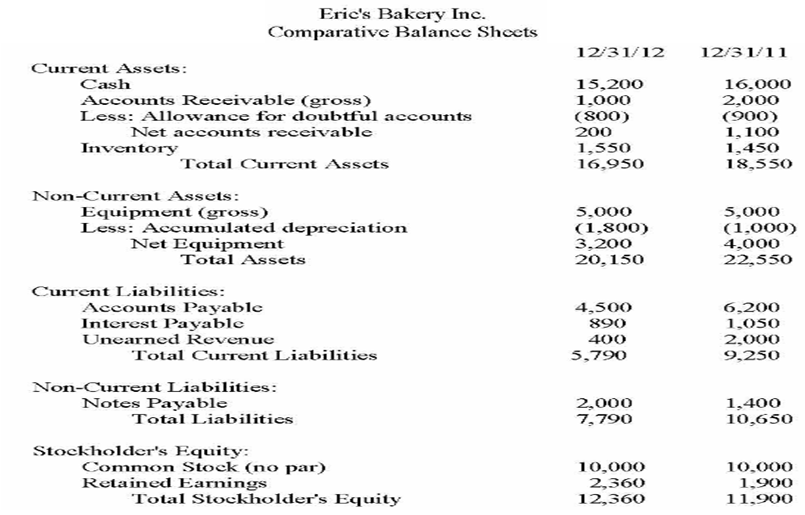# Problem: Given that Eric's total revenues for 2012 were $7,600 and net income for 2012 was$1,460, what was Eric's return on equity for 2012? A. 11.812% B. 37.717% C. 61.489 % D. 12.036%

###### Problem Details

Given that Eric's total revenues for 2012 were $7,600 and net income for 2012 was$1,460, what was Eric's return on equity for 2012?

A. 11.812%

B. 37.717%

C. 61.489 %

D. 12.036%